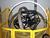## Converting AS/400 negative numeric to character with leading zerosTags:
AS/400
AS/400 numeric fields
iseries v5r4
Numeric conversion
I need to convert numeric values to character keeping the leading zeros and when the value is a negative, I need to prepend the negative sign in rpgle.

Example: Positive Amount : 00005679.05 = 5,769.05

Negative Amount: 00000-500.25 = -500.25

I've tried the editc with the 'X' edit code but i need the negative sign.

Software/Hardware used:
AS400 with os v5r4

Thanks. We'll let you know when a new response is added.

Try edit code P
<pre>
Edit code summary

Code Comma Zeros Sign
1 Y Y N
2 Y N N
3 N Y N
4 N N N

5-9 User-defined
A Y Y CR
B Y N CR
C N Y CR
D N N CR
J Y Y – (right)
K Y N –
L N Y –
M N N –
N Y Y – (left)
O Y N –
P N Y –
Q N N -</pre>

## Discuss This Question: 3 Replies

•••